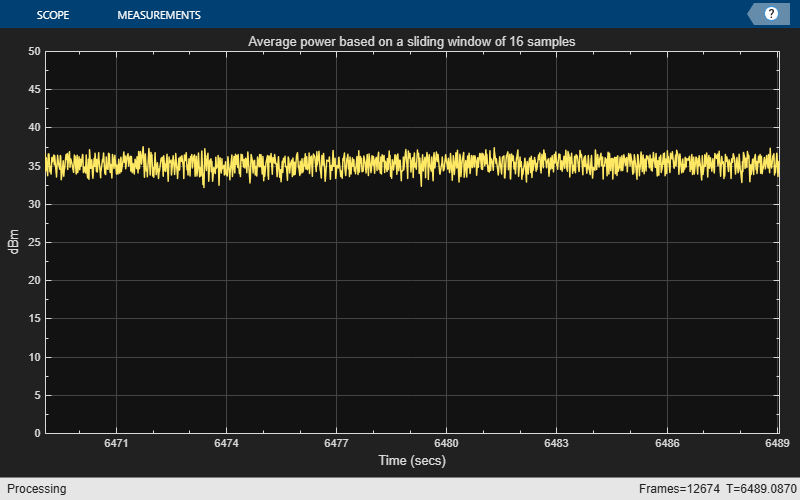# Compute Average Power of 256 QAM Signal in MATLAB

Compute the average power of a 256 QAM signal using a sliding window of 16 samples.

### Initialization

Create a `powermeter` object with a sliding window of length 16 samples and a reference load of 50 ohms. Use this object to measure the average power of a 256 QAM signal in `dBm` units.

Visualize the average power of the signal using the `timescope` object.

```FrameLength = 512; Fs = 1000; pm = powermeter(16, 'Measurement', 'Average power', ... 'ReferenceLoad', 50, ... 'PowerUnits', 'dBm'); scope = timescope('SampleRate',Fs,... 'TimeSpanSource','property',... 'TimeSpan',20,... 'YLabel','dBm',... 'YLimits',[0 50]); title = 'Average power based on a sliding window of 16 samples'; scope.Title = title;```

### Compute the Average Power

Generate a sequence of pseudorandom integer values drawn uniformly from [0 255] using the `randi` function. Apply quadrature amplitude modulation (QAM) on this signal using the `qammod` function. Compute the average power of this signal using the `powermeter` object. View the computed average using the `timescope` object.

```tic; while (toc < 5) x = randi([0 255], FrameLength, 1); y = qammod(x, 256); averagePower = pm(y); scope(averagePower); end```